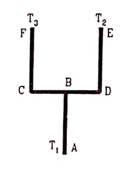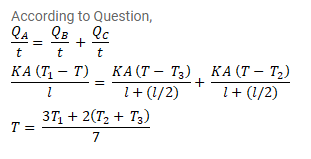# Four identical rods AB, CD, CF and DE are joined as shown in figure.Question:

Four identical rods $\mathrm{AB}, \mathrm{CD}, \mathrm{CF}$ and $\mathrm{DE}$ are joined as shown in figure. The length, cross - sectional area and thermal conductivity of each rod are $I, A$ and $K$ respectively. The ends $A, E$ and $F$ are maintained at temperatures $T_{1}, T_{2}$ and $T_{3}$ respectively. Assuming no loss of heat to the atmosphere, find the temperature at B.Solution: